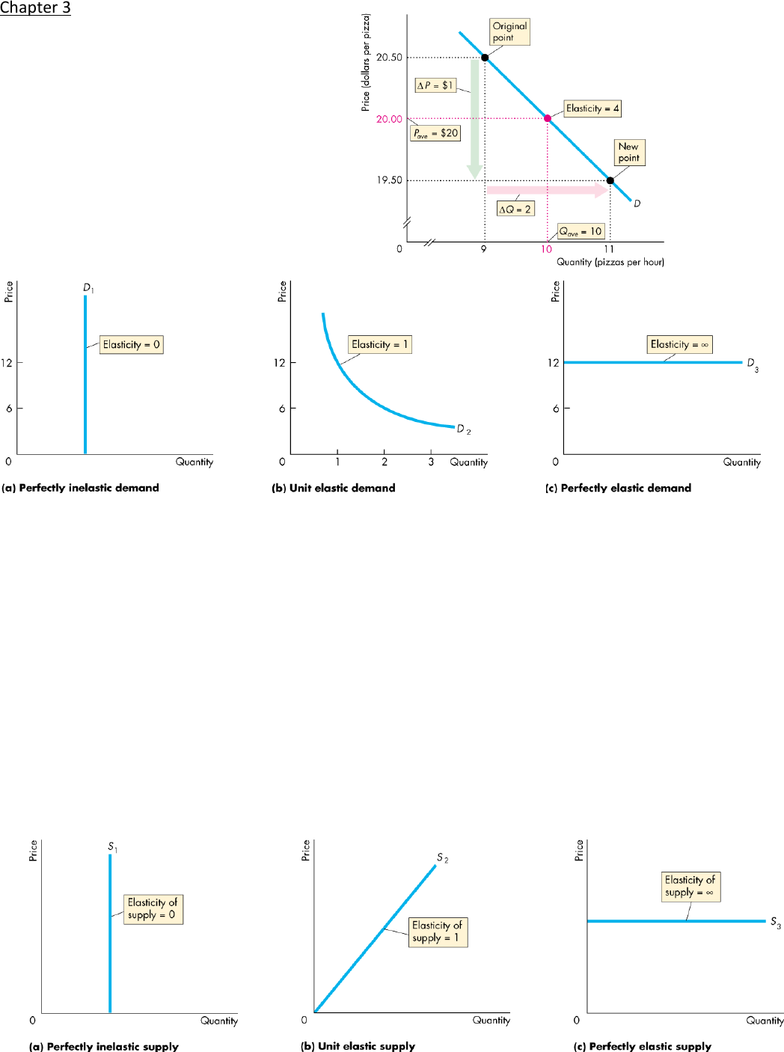Textbook Notes (270,000)
CA (160,000)
UW (6,000)
ECON (500)
ECON101 (200)
Chapter 3

# ECON101 Chapter Notes - Chapter 3: Demand Curve, Normal Good, Inferior Good

Department
Economics
Course Code
ECON101
Professor
Eva Lau
Chapter
3

Page:
of 2Chapter 3
- Price elasticity of demand is unit-free measure of
responsiveness of quantity demanded of good to
change in its price when everything else remains the
same
- Use value in middle of change as denominator when
calculating percentage to have more constant
numbers
- magnitude of price elasticity of demand tells us the
responsiveness of quantity demanded; take
absolute value
- demand becomes less elastic as price falls along linear demand curve
- total revenue test is method to estimate price elasticity of demand based on change in total revenue due to price change
o if price cut increases revenue, demand elastic
o if price cut decreases revenue, demand inelastic
o if price cut doesn’t change revenue, unit elastic
- factors that influence elasticity of demand are: closeness of substitutes, proportion of income spent on good, and time elapsed
since price change
o closeness of substitutes closer the substitutes are, more elastic the demand gasoline has no close substitutes, inelastic
demand Dell, HP, etc all close substitutes, elastic demand
o proportion of income spent on good greater the proportion, larger the elasticity of demand
o time since price change more time consumer has to adjust to price change or longer good can be stored without losing
value, more elastic the demand
- cross elasticity of demand measures responsiveness of demand for good to change in price of substitute or complement, other
things the same; cross elasticity of demand is positive for substitute and negative for complement
- income elasticity of demand measures how quantity demanded of goods responds to change in income, other things the same
- if income elasticity of demand >1, demand income elastic, and good is normal good; if <1, demand income inelastic, good normal; if
<0, good inferior good
- elasticity of supply measures responsiveness of quantity supplied to change in price of good when all other influences of selling
plans remain the same
E.g.
insuli
n
- factors that influence elasticity of supply are resource substation possibilities and time frame for supply decision
o resource substitution possibilities easier it is to substitute among resources used to produce good, greater its elasticity
of supply
o time frame for supply decisions more time that passes after price change, greater elasticity of supply; Momentary
supply is perfectly inelastic (quantity supplied immediately following price change constant); short-run supply somewhat
elastic; long run supply most elastic
TABLE 4.1 page 99